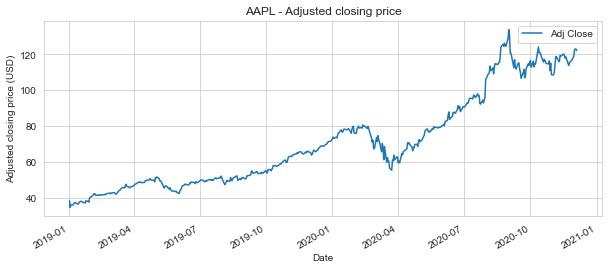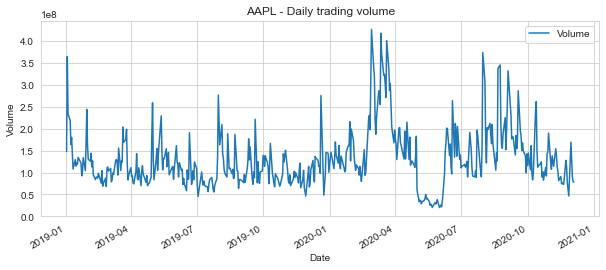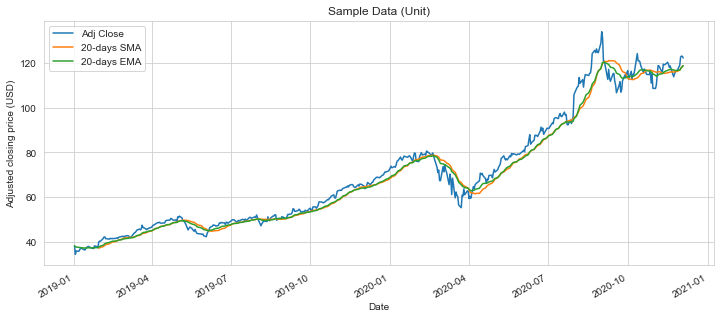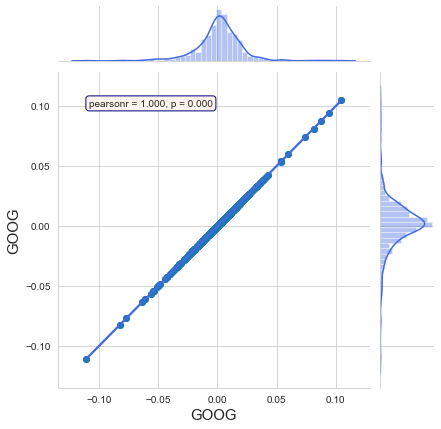# Stock Market Analysis (FAANG + Microsoft)¶

In this project, I will analyse stock market data for some technology stocks using Python. In particular, I will leverage various Python libraries (such as pandas, numpy, matplotlib and seaborn) to get stock data, create visualizations, analyze correlations, analyze risk, and predict future stock behavior.

I'll try to answer the following questions:

1.) What was the historical price changes for the stocks?
2.) What was the average daily return for the stocks?
3.) What was the moving average for the stocks?
4.) What was the correlation between the stocks' daily returns?
5.) How much value do we put at risk by investing in a particular stock?
6.) How can we attempt to predict future stock behavior?

# Section 1: Data preparation and basic analysis ¶

This sections include how to handle stock information with pandas, and how to do basic analysis of a stock's historical price and volume development.

## Section 1.1: Import required dependencies ¶

First, let’s import our required dependencies.

In :
#For data analysis
import pandas as pd
from pandas import Series,DataFrame
import numpy as np
# For visualization
import matplotlib.pyplot as plt
import seaborn as sns
sns.set_style('whitegrid')
#display graphs directly below the code cell that produced it
%matplotlib inline
# For stock data import
#conda install -c anaconda pandas-datareader #uncomment this line if you haven't installed pandas datareader
import pandas_datareader.data as web
# To show the correlation coefficient and p-value
import scipy.stats as stats
# For time stamps
from datetime import datetime, timedelta
# Required for Python version 2.x, will change the / operator to mean true division as implemented in Python 3.0.
#from __future__ import division #uncomment this line if you run Python 2.x


## Section 1.2: Download and Explore the Dataset ¶

Let's use Yahoo and pandas to grab some data for some tech stocks.

In :
# A list of the ticker symbols for the stocks I'll use for this analysis
tech_list = ['AAPL','GOOG','MSFT','AMZN','NFLX','FB']
# Set up End and Start times for data grab
#end_date = datetime.now()
end_date = datetime.now()
start_date = datetime(end_date.year - 1,month=1, day=1)
print(f'start date = {start_date}')
print(f'end date = {end_date}')
#For loop for grabing yahoo finance data and setting as a dataframe
for stock in tech_list:
# Set DataFrame as the Stock Ticker

start date = 2019-01-01 00:00:00
end date = 2020-12-05 23:41:44.314769


Let's have a quick look at the AAPL DataFrame

In :
AAPL.head().round(decimals=2)

Out:
Date
2019-01-0239.7138.5638.7239.48148158800.038.25
2019-01-0336.4335.5035.9935.55365248800.034.44
2019-01-0437.1435.9536.1337.06234428400.035.91
2019-01-0737.2136.4737.1736.98219111200.035.83
2019-01-0837.9637.1337.3937.69164101200.036.51

The adjusted closing price ("Adj Close") amends a stock's closing price to reflect that stock's value after accounting for any corporate actions. It is often used when examining historical returns or doing a detailed analysis of past performance (Source: https://www.investopedia.com/terms/a/adjusted_closing_price.asp).

Let's apply the describe function to get some basic statistical details like percentiles, mean, std etc.

In :
# Summary statistics
AAPL.describe().round(decimals=2)

Out:
count487.00487.00487.00487.004.870000e+02487.00
mean72.5670.7971.6471.731.307927e+0870.86
std26.1025.1525.7125.636.646178e+0726.01
min36.4335.5035.9935.552.019780e+0734.44
25%51.0250.1850.5450.678.891020e+0749.43
50%66.5265.2765.9266.041.144304e+0864.94
75%88.0385.0387.1586.711.558620e+0886.41
max137.98130.53137.59134.184.268848e+08133.95
In :
# General Info
AAPL.info()

<class 'pandas.core.frame.DataFrame'>
DatetimeIndex: 487 entries, 2019-01-02 to 2020-12-04
Data columns (total 6 columns):
#   Column     Non-Null Count  Dtype
---  ------     --------------  -----
0   High       487 non-null    float64
1   Low        487 non-null    float64
2   Open       487 non-null    float64
3   Close      487 non-null    float64
4   Volume     487 non-null    float64
5   Adj Close  487 non-null    float64
dtypes: float64(6)
memory usage: 26.6 KB


## Section 1.3: Plot out the volume and closing price ¶

Now that we've seen the DataFrame, let's go ahead and plot out the volume and closing price of the stocks

In :
# Let's see a historical view of the closing price
# Add appropriate axis labels

Out:
[Text(0, 0.5, 'Adjusted closing price (USD)'), Text(0.5, 0, 'Date')]Now that we've seen the development of the adjusted closing price, let's plot the Daily volume (i.e. how many shares are traded each day).

In :
# Now let's plot the daily volume, i.e. how many AAPL shares are traded each day
AAPL_vol = AAPL['Volume'].plot(legend=True,figsize=(10,4),title="AAPL - Daily trading volume")
# Add appropriate axis labels
AAPL_vol.set(xlabel="Date", ylabel="Volume")

Out:
[Text(0, 0.5, 'Volume'), Text(0.5, 0, 'Date')]The Daily volume can be averaged over a number of days (typically over 20 or 30 days) to find the average daily trading volume (ADTV), i.e. the average number of shares traded within a day in a given stock. ADTV is an important metric because high or low trading volume attracts different types of traders and investors. Many traders and investors prefer higher ADTV compared to low trading volume, because with high volume it is easier to get into and out positions. Low volume assets have fewer buyers and sellers, and therefore it may be harder to enter or exit at a desired price (Source: https://www.investopedia.com/terms/a/averagedailytradingvolume.asp).

In :
# Let's add a 30 days Average Daily Trading Volume metric
AAPL.tail().round(decimals=2)

Out:
Date
2020-11-30120.97116.81116.97119.05169410200.0119.051.108967e+08
2020-12-01123.47120.01121.01122.72128166800.0122.721.111476e+08
2020-12-02123.37120.89122.02123.0889004200.0123.081.099670e+08
2020-12-03123.78122.21123.52122.9478967600.0122.941.096010e+08
2020-12-04122.86121.52122.60122.2578133200.0122.251.088059e+08
In :
# Now let's plot the daily volume, i.e. how many AAPL shares are traded each day
AAPL_vol_avg = AAPL[['Volume','ADTV']].plot(legend=True,figsize=(10,4),title="AAPL - Daily trading volume")
# Add appropriate axis labels
AAPL_vol_avg.set(xlabel="Date", ylabel="Volume")

Out:
[Text(0, 0.5, 'Volume'), Text(0.5, 0, 'Date')]## Section 1.4: Moving Average ¶

Now that we've seen the visualizations for the closing price and the volume traded each day, let's go ahead and caculate the moving average for the stock.

• A moving average (MA) is a stock indicator that is commonly used in technical analysis.
• The reason for calculating the moving average of a stock is to help smooth out the price data (i.e. by filtering out the “noise” from random short-term price fluctuations) over a specified period of time by creating a constantly updated average price.
• MAs can be constructed in several different ways, and employ different numbers of days for the averaging interval. The most common time periods used in moving averages are 15, 20, 30, 50, 100, and 200 days. The shorter the time span used to create the average, the more sensitive it will be to price changes. The longer the time span, the less sensitive the average will be. The 50-day and 200-day moving average figures for stocks are widely followed by investors and traders and are considered to be important trading signals.
• The most common applications of MAs are to identify trend direction and to determine support and resistance levels. When asset prices cross over their MAs, it may generate a trading signal for technical traders
• A simple moving average (SMA) is a calculation that takes the arithmetic mean of a given set of prices over the specific number of days in the past; for example, over the previous 15, 30, 100, or 200 days.
• Exponential moving averages (EMA) is a weighted average that gives greater importance to the price of a stock on more recent days, making it an indicator that is more responsive to new information. If you plot a 50-day SMA and a 50-day EMA on the same chart, you'll notice that the EMA reacts more quickly to price changes than the SMA does, due to the additional weighting on recent price data.

Sources:

#### Simple moving average (SMA)¶

In :
# Let's go ahead and plot out several moving averages
ma_day = [20,50,200]
for ma in ma_day:
column_name = "%s-days SMA" %(str(ma))

AAPL[column_name] = AAPL['Adj Close'].rolling(window=ma,center=False).mean()

In :
AAPL.tail().round(decimals=2)

Out:
Date
2020-11-30120.97116.81116.97119.05169410200.0119.051.108967e+08116.79115.7093.97
2020-12-01123.47120.01121.01122.72128166800.0122.721.111476e+08117.49115.9694.19
2020-12-02123.37120.89122.02123.0889004200.0123.081.099670e+08118.14116.1994.40
2020-12-03123.78122.21123.52122.9478967600.0122.941.096010e+08118.54116.5194.62
2020-12-04122.86121.52122.60122.2578133200.0122.251.088059e+08118.72116.8094.85

Now let's go ahead and plot all the additional Moving Averages

In :
ax = AAPL[['Adj Close','20-days SMA','50-days SMA','200-days SMA']].plot(subplots=False,figsize=(12,5),title="Sample Data (Unit)")
ax.set(xlabel="Date", ylabel="Adjusted closing price (USD)")

Out:
[Text(0, 0.5, 'Adjusted closing price (USD)'), Text(0.5, 0, 'Date')]It is straightforward to observe that SMA timeseries are much less noisy than the original price timeseries. However, this comes at a cost. SMA timeseries lag the original price timeseries, which means that changes in the trend are only seen with a delay (lag) of L days.

How much is this lag L? For a SMA moving average calculated using M days, the lag is roughly M/2 days. Thus, if we are using a 100 days SMA, this means we may be late by almost 50 days, which can significantly affect our strategy.

#### Exponential Moving Average (EMA)¶

One way to reduce the lag induced by the use of the SMA is to use the so-called Exponential Moving Average (EMA). The reason why EMA reduces the lag is that it puts more weight on more recent observations, whereas the SMA weights all observations equally by 1/M. Using Pandas, calculating the exponential moving average is easy. We need to provide a lag value, from which the decay parameter α is automatically calculated.

In :
# Let's go ahead and plot out several moving averages
# Span (i.e. the length of the window) corresponds to what is commonly called an “N-day EW moving average”.
AAPL.tail().round(decimals=2)

Out:
Date
2020-11-30120.97116.81116.97119.05169410200.0119.051.108967e+08116.79115.7093.97116.87
2020-12-01123.47120.01121.01122.72128166800.0122.721.111476e+08117.49115.9694.19117.42
2020-12-02123.37120.89122.02123.0889004200.0123.081.099670e+08118.14116.1994.40117.96
2020-12-03123.78122.21123.52122.9478967600.0122.941.096010e+08118.54116.5194.62118.44
2020-12-04122.86121.52122.60122.2578133200.0122.251.088059e+08118.72116.8094.85118.80
In :
ax = AAPL[['Adj Close','20-days SMA','20-days EMA']].plot(subplots=False,figsize=(12,5),title="Sample Data (Unit)")
ax.set(xlabel="Date", ylabel="Adjusted closing price (USD)")

Out:
[Text(0, 0.5, 'Adjusted closing price (USD)'), Text(0.5, 0, 'Date')]In the graph above, the number of time periods used in each average is identical–20–but the EMA responds more quickly to the changing prices than the SMA. You can also observe in the figure that the EMA has a higher value when the price is rising than the SMA (and it falls faster than the SMA when the price is declining). This responsiveness to price changes is the main reason why some traders prefer to use the EMA over the SMA (source: http://www.investopedia.com/terms/m/movingaverage.asp).

In [ ]:



# Section 2: Daily return & correlation Analysis ¶

Now that we've done some baseline analysis, let's go ahead and dive a little deeper. We're now going to analyze the risk of the stock. In order to do so we'll need to take a closer look at the daily changes of the stock, and not just its absolute value. Let's go ahead and use pandas to retrieve the daily returns for the Apple stock.

In :
# The daily return column can be created by using the percentage change for the adjusted closing price
AAPL['Daily Return'] = AAPL['Adj Close'].pct_change()

Out:
Date
2019-01-02         NaN
2019-01-03   -0.099607
2019-01-04    0.042689
2019-01-07   -0.002226
2019-01-08    0.019063
Name: Daily Return, dtype: float64
In :
# Then we'll plot the daily return percentage
ax = AAPL['Daily Return'].plot(figsize=(14,5),legend=True,linestyle='--',marker='o',title="AAPL - Daily return percentage")
ax.set(xlabel='Date', ylabel='Daily percentage change')

Out:
[Text(0, 0.5, 'Daily percentage change'), Text(0.5, 0, 'Date')]Great, now let's get an overall look at the average daily return using a histogram. We'll use seaborn to create both a histogram and kernel density estimate (KDE) plot on the same figure. A KDE plot is a method for visualizing the distribution of observations in a dataset. KDE represents the data using a continuous probability density curve in one or more dimensions.

In :
# Note the use of dropna() here, otherwise the NaN values can't be read by seaborn
ax = sns.distplot(AAPL['Daily Return'].dropna(),bins=100,color='blue')

ax.set(xlabel='Daily return', ylabel='# of observations',title="AAPL - Daily return distribution")
# Could have also done:
#AAPL['Daily Return'].hist()

Out:
[Text(0, 0.5, '# of observations'),
Text(0.5, 0, 'Daily return'),
Text(0.5, 1.0, 'AAPL - Daily return distribution')]Positive daily returns seem to be slightly more frequent than negative returns for Apple, which makes sense given the historical development of the closing price.

We will make the assumption that the daily returns is normally distributed. Normally distributed variables have a bell-shaped curve which is symmetrical (i.e. half of the data will fall to the left of the mean and half will fall to the right).

## Section 2.1: Correlation between the stocks' daily returns ¶

Now what if we wanted to analyze the returns of all the stocks in our list? Let's go ahead and build a DataFrame with all the ['Adj Close'] columns for each of the stocks dataframes.

In :
# Grab all the closing prices for the tech stock list into one DataFrame

In :
# Let's have a quick look
closing_df.tail().round(decimals=2)

Out:
SymbolsAAPLGOOGMSFTAMZNNFLXFB
Date
2020-11-30119.051760.74214.073168.04490.70276.97
2020-12-01122.721798.10216.213220.08504.58286.55
2020-12-02123.081827.95215.373203.53503.38287.52
2020-12-03122.941826.77214.243186.73497.52281.85
2020-12-04122.251827.99214.363162.58498.31279.70

Now that we have all the historical closing prices for the stocks in the same dataframe, we can use Pandas’ pct_change method to calculate the daily returns for each stock (just as we did earlier with Apple).

In :
# Add a new returns DataFrame
rets_df = closing_df.pct_change()
rets_df.tail()

Out:
SymbolsAAPLGOOGMSFTAMZNNFLXFB
Date
2020-11-300.021100-0.018096-0.005390-0.008544-0.001343-0.003024
2020-12-010.0308270.0212180.0099970.0164270.0282860.034589
2020-12-020.0029340.016601-0.003885-0.005140-0.0023780.003385
2020-12-03-0.001137-0.000645-0.005247-0.005244-0.011641-0.019720
2020-12-04-0.0056130.0006680.000560-0.0075780.001588-0.007628

Now we can move on to analyse the correlation between the stocks daily returns. We will calculate a correlation coefficient to measure the correlation between two stocks.

Correlation coefficients are used in statistics to measure how strong a relationship is between two variables. There are several types of correlation coefficient, but the most popular is Pearson’s. Pearson correlation coefficient (PCC), also referred to as Pearson's r, is a statistic that measures linear correlation between two variables. It has a value between +1 and −1. A value of +1 equals a total positive linear correlation, 0 is no linear correlation, and −1 is total negative linear correlation.

For the Pearson r correlation, both variables should be normally distributed. We have already made the assumption that the daily returns for Apple is normally distributed, and we will make the same assumption for the remainder of the stocks.

In :
# Examples of scatter diagrams with different values of correlation coefficient (ρ)
from IPython.display import Image
from IPython.core.display import HTML
Image(url= "https://upload.wikimedia.org/wikipedia/commons/3/34/Correlation_coefficient.png", width=500, height=500)

Out:Visit https://en.wikipedia.org/wiki/Pearson_correlation_coefficient for further information about Pearson’s.

First let's see a stock compared to itself. Let's look at Google.

In :
# Let's filter the rets_df to only show the column we're interested in
rets_df['GOOG']

Out:
Date
2019-01-02         NaN
2019-01-03   -0.028484
2019-01-04    0.053786
2019-01-07   -0.002167
2019-01-08    0.007385
...
2020-11-30   -0.018096
2020-12-01    0.021218
2020-12-02    0.016601
2020-12-03   -0.000645
2020-12-04    0.000668
Name: GOOG, Length: 487, dtype: float64

When comparing a stock to itself it should show a perfectly linear relationship

In :
# Code to build a Scatter Plot with Marginal Histograms
stocks = {'a':'GOOG','b':'GOOG'} #Add the tickers for the two stocks you want to compare
a = rets_df[stocks['a']].dropna()
b = rets_df[stocks['b']].dropna()
r, p = stats.pearsonr(a, b)
#print("Pearson’s correlation coefficient is {}".format(np.corrcoef(a, b)))
print(f'Pearson’s correlation coefficient (PCC) is {r:.3f}, and the p-value is {p:.3f}')
g = sns.jointplot(x=a, y=b, kind='reg', color='royalblue')
#r, p = stats.pearsonr(a, b)
g.ax_joint.annotate(f'pearsonr = {r:.3f}, p = {p:.3f}',
xy=(0.1, 0.9), xycoords='axes fraction',
ha='left', va='center',
bbox={'boxstyle': 'round', 'fc': 'seashell', 'ec': 'navy'})
g.ax_joint.scatter(a, b)
g.set_axis_labels(xlabel=stocks['a'], ylabel=stocks['b'], size=15)
#plt.tight_layout()
#plt.show()

Pearson’s correlation coefficient (PCC) is 1.000, and the p-value is 0.000

Out:
<seaborn.axisgrid.JointGrid at 0x7f8e85d84520>Here's a suggested user's guide for interpreting the absolute value of r:

• .00-.19 “very weak”
• .20-.39 “weak”
• .40-.59 “moderate”
• .60-.79 “strong”
• .80-1.0 “very strong”

The graph shows, as expected, a perfectly linear relationship with a PCC of +1. A correlation value of r=1.0 would be a "very strong" positive correlation according to the user guide above.

Let's instead look at the daily returns for two different stocks. Let's look at Google and Apple.

In :
# Code to build a Scatter Plot with Marginal Histograms
stocks = {'a':'GOOG','b':'AAPL'} #Add the tickers for the two stocks you want to compare
a = rets_df[stocks['a']].dropna()
b = rets_df[stocks['b']].dropna()
r, p = stats.pearsonr(a, b)
#print("Pearson’s correlation coefficient is {}".format(np.corrcoef(a, b)))
print(f'Pearson’s correlation coefficient (PCC) is {r:.3f}, and the p-value is {p:.3f}')
g = sns.jointplot(x=a, y=b, kind='reg', color='royalblue')
#r, p = stats.pearsonr(a, b)
g.ax_joint.annotate(f'pearsonr = {r:.3f}, p = {p:.3f}',
xy=(0.1, 0.9), xycoords='axes fraction',
ha='left', va='center',
bbox={'boxstyle': 'round', 'fc': 'seashell', 'ec': 'navy'})
g.ax_joint.scatter(a, b)
g.set_axis_labels(xlabel=stocks['a'], ylabel=stocks['b'], size=15)
#plt.tight_layout()
#plt.show()

Pearson’s correlation coefficient (PCC) is 0.705, and the p-value is 0.000

Out:
<seaborn.axisgrid.JointGrid at 0x7f8e7f084df0>Google and Apple appears to have a quite strong positive correlation in terms of daily percentage returns. As seen we got a pearsonr of 0.7. Let's have another look at the suggested user's guide for interpreting the absolute value of r:

• .00-.19 “very weak”
• .20-.39 “weak”
• .40-.59 “moderate”
• .60-.79 “strong”
• .80-1.0 “very strong”

A correlation value of r=0.7 would be a "strong" positive correlation.

Seaborn and pandas make it very easy to repeat this comparison analysis for every possible combination of stocks in our technology stock ticker list. We can use sns.pairplot() to automatically create this plot

In :
sns.pairplot(rets_df.dropna())

Out:
<seaborn.axisgrid.PairGrid at 0x7f8e86e0f550>Pairs plots are a powerful tool to quickly explore distributions and relationships in a dataset. Seaborn provides a simple default method for making pair plots that can be customized and extended through the Pair Grid class.

In contrast to the sns.pairplot function, sns.PairGrid is a class which means that it does not automatically fill in the plots for us. Instead we get full control of the figure. It lets us create and map specific functions to the different sections of the grid (i.e. the diagonal, the upper triangle, and the lower triangle). This is the real benefit of using the PairGrid. For example, we might want to add the PCC between each pair of stocks in the scatterplot. Below is an example of utilizing the full power of seaborn.

In :
# Function to calculate correlation coefficient between two arrays
def corr(x, y, **kwargs):

# Calculate the value
coef = np.corrcoef(x, y)
# Make the label
label = r'$\rho$ = ' + str(round(coef, 3))

# Add the label to the plot
ax = plt.gca()
ax.annotate(label, xy = (0.2, 0.95), size = 15, xycoords = ax.transAxes)
# Set up our figure by naming it returns_fig, call PairPLot on the DataFrame
returns_fig = sns.PairGrid(rets_df.dropna(), diag_sharey=False, corner=True)
# Using map_upper we can specify what the upper triangle will look like.
#returns_fig.map_upper(plt.scatter,color='seagreen')
#returns_fig.map_upper(corr)
# We can also define the lower triangle in the figure, inclufing the plot type (kde) or the color map (BluePurple)
returns_fig.map_lower(sns.regplot, color='seagreen', scatter_kws={'s':15})
returns_fig.map_lower(corr)
# Finally we'll define the diagonal as a series of histogram plots of the daily return
returns_fig.map_diag(plt.hist,bins=30)

Out:
<seaborn.axisgrid.PairGrid at 0x7f8e88184580>